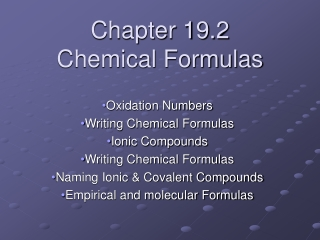DownloadDownload PresentationChapter 19.2 Chemical Formulas

# Chapter 19.2 Chemical Formulas

Télécharger la présentation## Chapter 19.2 Chemical Formulas

- - - - - - - - - - - - - - - - - - - - - - - - - - - E N D - - - - - - - - - - - - - - - - - - - - - - - - - - -
##### Presentation Transcript

1. Chapter 19.2 Chemical Formulas Oxidation Numbers Writing Chemical Formulas Ionic Compounds Writing Chemical Formulas Naming Ionic & Covalent Compounds Empirical and molecular Formulas

2. Oxidation Numbers • An oxidation number indicates how many electrons will be lost, gained or shared when bonding occurs • ALL COMPOUNDS have an electrical charge of ZERO • When the oxidation numbers are added up for all of the atoms in a compound, the answer equals zero

3. Oxidation Numbers & the Periodic Table • You can use the periodic table to predict the oxidation numbers for some of the groups. • The transition metals are an exception, they have variable oxidation numbers • Periodic Table

4. Writing Chemical Formulas for Ionic compounds • Write the symbol for the positive . monatomic ion. (Groups 1, 2, & 13) • Write the symbol for the negative monatomic ion. (Groups 15, 16, & 17) • Add superscripts so that the sum of the oxidation numbers equals zero

5. Writing a Chemical Formula • CrissCross Applesauce is all you need to know

6. Ionic CompoundsPolyatomic Ions • Most Ionic compounds are made up of more than 2 types of atoms called Polyatomic Ions. (see table on p. 337) • Treat them just as you would when working with monatomic ions, except you need to put the polyatomic ion in brackets and place the oxidation number as a superscript on the outside of the bracket.

7. Naming monatomic Ionic Compounds • Write the root name of the second element • Add “ide” to the root name • Write the name of the first element in the compound Ex: Na + Cl = NaCl, Naming this chemical formula would be , Sodium plus Chlorine equals Sodium chloride.

8. Naming Compounds with Polyatomic Ions • Write the name of the positive ion first • Write the name of the negative ion second You can use the periodic table or an ion chart to get the names- There is one on p. 337 of your text book. Treat them just as you would the monatomic ions.

9. Empirical Formula • The simplest whole number ratio by which the elements combine. Ex: Hydrogen peroxide, each molecule contains 2 hydrogen and two oxygen atoms, but the empirical formula is HO

10. Molecular Formula • A molecular formula contains the actual number of atoms for each element in one molecule of the compound Ex: Hydrogen peroxide, each molecule contains 2 hydrogen and two oxygen atoms, and the molecular formula is H2O2